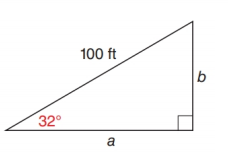Chapter 11.2, Problem 17EElementary Geometry For College St...

7th Edition
Alexander + 2 others
ISBN: 9781337614085

Solutions

Chapter
SectionElementary Geometry For College St...

7th Edition
Alexander + 2 others
ISBN: 9781337614085
Textbook Problem

In Exercise 17 to 22, use either the sine ratio or the cosine ratio to find the length of the indicated sides of the triangle correct to the nearest tenth of a unit.To determine

To find:

The length of the indicated sides of the following triangle,Explanation

Consider the following figure,

General formula for cosine ratio and sin ratio is given below,

From the given figure the value 100 ft is the hypotenuse value since it is opposite to right angle.

Then a is the length of the leg adjacent to 32 and b is the length of leg opposite to 32.

Therefore,

cos32=a100a=100×cos32

Using a scientific calculator in degree mode, follow the following key sequence,

32cos0

Still sussing out bartleby?

Check out a sample textbook solution.

See a sample solution

The Solution to Your Study Problems

Bartleby provides explanations to thousands of textbook problems written by our experts, many with advanced degrees!

Get Started

Evaluate the integrals in Problems 1-26.

Mathematical Applications for the Management, Life, and Social Sciences

In Exercises 1-10, determine which of the matrices are stochastic. 

Finite Mathematics for the Managerial, Life, and Social Sciences

What is the integrating factor for xy′ + 6x2y = 10 − x3?

Study Guide for Stewart's Single Variable Calculus: Early Transcendentals, 8th